Related Tags

project euler
maximum path sum i
communitycreator

# How to solve Project Euler #18 - maximum path sum problem

Armaan Nougai

### Maximum path sum

Starting from the top of the number’s triangle and moving to adjacent numbers on the row below, find the maximum total from top to bottom of the given triangles.

18th Problem triangle
67th Problem triangle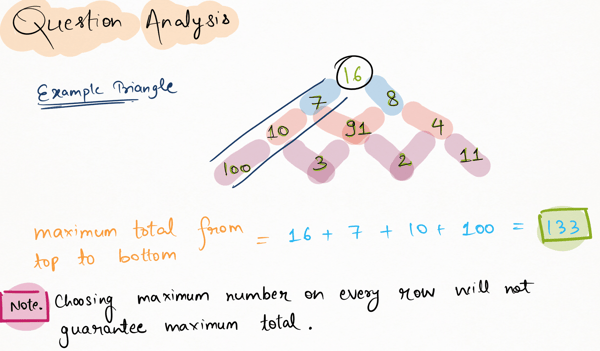### Brute force approach

• Storing the triangle - We will accept the triangle text input, and will convert it into a list of rows, where a row is the list of elements.

• Algorithm - For each row, we will calculate an array res_array from top to bottom. This array will consist of the sums of the numbers of the best path from the top to the respective elements in that row.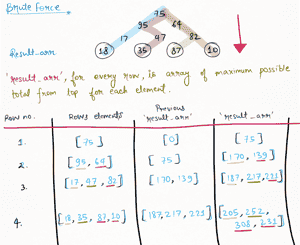• Note: The code below is for problems 18 and 67.

First, paste the triangle in the input box to run the code.

# Coded By: Armaan Nougai
#Brute Force
# Project Euler 18 and 67

with open('__ed_input.txt','r') as FILE:
arr = [list(map(int,(j.split()))) for j in Data]

result_arr = arr
for row in arr[1:]:
new_result_arr = []
for x,value in enumerate(row):
if x==0:
# 1st element of row
new_result_arr += [value+result_arr]
elif x==len(row)-1:
# last element of row
new_result_arr += [value + result_arr[-1]]
else:
# mid-elements of row
new_result_arr += [value+max(result_arr[x],result_arr[x-1])]

result_arr = new_result_arr

print(max(result_arr))


Enter the input below to be saved in file __ed_input.txt

### Better approach

• We’ll store the triangle in a list of rows, where a row is a list of elements.

• Algorithm -

• Let the figure below be the triangle

 75
95 64
17 47 82
18 35 87 10
20 04 82 47 65

1. We will start from the second to last row, and create a res_array, as follows:

• For 18: we have two options, 20 and 04, and we will choose the larger one, i.e. 20. So res_array= 18+20= 38

• For 35: we’ll choose max(04,82) = 82. res_array= 35+82=117

• For 87: we’ll choose max(82,47) = 82. res_array=87+82=169

• For 10: we’ll choose max(47,65) = 65. res_array=10+65=75.

And now, we will replace the last two rows of the triangle with res_array.

1. Then, we will again go through the second to last row and create res_array.

• res_array=17+117=134

• res_array=47+169=216

• res_array=82+169=251

Again, we will replace the last two rows of the triangle with res_array.

1. We will repeat the steps above until the res_array has only one element in it.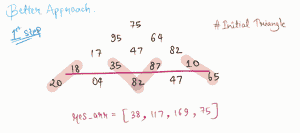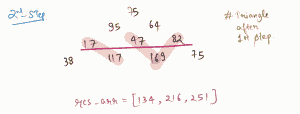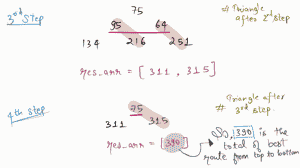# Coded By: Armaan Nougai
# Project Euler 18 and 67

with open('__ed_input.txt','r') as FILE:
arr = [list(map(int,(j.split()))) for j in Data]

result_arr = *(len(arr[-1])+1)
for row in arr[::-1]:

result_arr = list([value + max(result_arr[x],result_arr[x+1]) for x,value in enumerate(row)])

print(result_arr)

Enter the input below to be saved in file __ed_input.txt

RELATED TAGS

project euler
maximum path sum i
communitycreator

CONTRIBUTOR

Armaan Nougai
RELATED COURSES

View all Courses

Keep Exploring

Learn in-demand tech skills in half the time